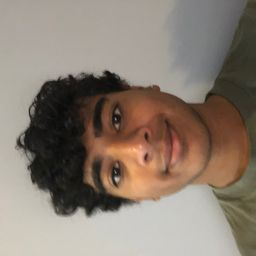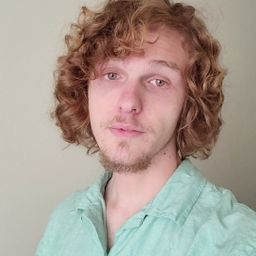## Educators### Problem 1

Let $g(x, y)=\cos (x+2 y)$
(a) Evaluate $g(2,-1)$
(b) Find the domain of $g .$
(c) Find the range of $g .$### Problem 2

Let $F(x, y)=1+\sqrt{4-y^{2}}$
(a) Evaluate $F(3,1)$ .
(b) Find and sketch the domain of $F .$
(c) Find the range of $F .$### Problem 3

Let $f(x, y, z)=\sqrt{x}+\sqrt{y}+\sqrt{z}+\ln \left(4-x^{2}-y^{2}-z^{2}\right)$
(a) Evaluate $f(1,1,1)$ .
(b) Find and describe the domain of $f .$### Problem 4

Let $g(x, y, z)=x^{3} y^{2} z \sqrt{10-x-y-z}$
(a) Evaluate $g(1,2,3)$ .
(b) Find and describe the domain of $g .$### Problem 5

Find and sketch the domain of the function.
$$f(x, y)=\sqrt{2 x-y}$$### Problem 6

Find and sketch the domain of the function.
$$f(x, y)=\sqrt{x y}$$### Problem 7

Find and sketch the domain of the function.
$$f(x, y)=\ln \left(9-x^{2}-9 y^{2}\right)$$### Problem 8

Find and sketch the domain of the function.
$$f(x, y)=\sqrt{y}+\sqrt{25-x^{2}-y^{2}}$$### Problem 9

Find and sketch the domain of the function.
$$f(x, y)=\frac{\sqrt{y-x^{2}}}{1-x^{2}}$$### Problem 10

Find and sketch the domain of the function.
$$f(x, y)=\arcsin \left(x^{2}+y^{2}-2\right)$$### Problem 11

Find and sketch the domain of the function.
$$f(x, y, z)=\sqrt{1-x^{2}-y^{2}-z^{2}}$$### Problem 12

Find and sketch the domain of the function.
$$f(x, y, z)=\ln \left(16-4 x^{2}-4 y^{2}-z^{2}\right)$$### Problem 13

Sketch the graph of the function.
$$f(x, y)=10-4 x-5 y$$### Problem 14

Sketch the graph of the function.
$$f(x, y)=2-x$$### Problem 15

Sketch the graph of the function.
$$f(x, y)=y^{2}+1$$### Problem 16

Sketch the graph of the function.
$$f(x, y)=e^{-y}$$### Problem 17

Sketch the graph of the function.
$$f(x, y)=9-x^{2}-9 y^{2}$$### Problem 18

Sketch the graph of the function.
$$f(x, y)=1+2 x^{2}+2 y^{2}$$

Check back soon!

### Problem 19

Sketch the graph of the function.
$$f(x, y)=\sqrt{4-4 x^{2}-y^{2}}$$### Problem 20

Sketch the graph of the function.
$$f(x, y)=\sqrt{4 x^{2}+y^{2}}$$### Problem 21

A contour map for a function $f$ is shown. Use it to estimate the values of $f(-3,3)$ and $f(3,-2) .$ What can you say about the shape of the graph?Deven G.

### Problem 22

Two contour maps are shown. One is for a function $f$ whose graph is a cone. The other is for a function $g$ whose graph is a paraboloid. Which is which, and why?

Check back soon!

### Problem 23

Locate the points $A$ and $B$ on the map of Lonesome Mountain (Figure 11). How would you describe the terrain near $A$ ? Near $B ?$

Check back soon!

### Problem 24

Make a rough sketch of a contour map for the function whose graph is shown.

Check back soon!

### Problem 25

Draw a contour map of the function showing several level curves.
$$f(x, y)=(y-2 x)^{2}$$### Problem 26

Draw a contour map of the function showing several level curves.
$$f(x, y)=x^{3}-y$$Deven G.

### Problem 27

Draw a contour map of the function showing several level curves.
$$f(x, y)=\sqrt{x}+y$$

Check back soon!

### Problem 28

Draw a contour map of the function showing several level curves.
$$f(x, y)=\ln \left(x^{2}+4 y^{2}\right)$$

Check back soon!

### Problem 29

Draw a contour map of the function showing several level curves.
$$f(x, y)=y e^{x}$$### Problem 30

Draw a contour map of the function showing several level curves.
$$f(x, y)=y \sec x$$

Check back soon!

### Problem 31

Draw a contour map of the function showing several level curves.
$$f(x, y)=\sqrt{y^{2}-x^{2}}$$Deven G.

### Problem 32

Draw a contour map of the function showing several level curves.
$$f(x, y)=y /\left(x^{2}+y^{2}\right)$$

Check back soon!

### Problem 33

Sketch both a contour map and a graph of the function and compare them.
$$f(x, y)=x^{2}+9 y^{2}$$

Check back soon!

### Problem 34

Sketch both a contour map and a graph of the function and compare them.
$$f(x, y)=\sqrt{36-9 x^{2}-4 y^{2}}$$

Check back soon!

### Problem 35

A thin metal plate, located in the $x y$ -plane, has temperature $T(x, y)$ at the point $(x, y) .$ The level curves of $T$ are called isothermals because at all points on such a curve the temperature is the same. Sketch some isothermals if the temperature function is given by

Check back soon!

### Problem 35

A thin metal plate, located in the $x y$ -plane, has temperature $T(x, y)$ at the point $(x, y) .$ The level curves of $T$ are called isothermals because at all points on such a curve the temperature is the same. Sketch some isothermals if the temperature function is given by
$$T(x, y)=\frac{100}{1+x^{2}+2 y^{2}}$$Deven G.

### Problem 36

If $V(x, y)$ is the electric potential at a point $(x, y)$ in the $x y$ -plane, then the level curves of $V$ are called equipotential curves because at all points on such a curve the elec- tric potential is the same. Sketch some equipotential curves if $V(x, y)=c / \sqrt{r^{2}-x^{2}-y^{2}},$ where $c$ is a positive constant.Deven G.

### Problem 37

Use a computer to graph the function using various domains and viewpoints. Get a printout of one that, in your opinion, gives a good view. If your software also produces, level curves, then plot some contour lines of the same function and compare with the graph.
$$f(x, y)=x y^{2}-x^{3} \quad(monkey saddle)$$### Problem 38

Use a computer to graph the function using various domains and viewpoints. Get a printout of one that, in your opinion, gives a good view. If your software also produces, level curves, then plot some contour lines of the same function and compare with the graph.
$$f(x, y)=x y^{3}-y x^{3} \quad(dog saddle )$$Deven G.

### Problem 39

Use a computer to graph the function using various domains and viewpoints. Get a printout of one that, in your opinion, gives a good view. If your software also produces, level curves, then plot some contour lines of the same function and compare with the graph.
$$f(x, y)=e^{-\left(x^{2}+y^{3}\right) / 3}\left(\sin \left(x^{2}\right)+\cos \left(y^{2}\right)\right)$$Deven G.

### Problem 40

Use a computer to graph the function using various domains and viewpoints. Get a printout of one that, in your opinion, gives a good view. If your software also produces, level curves, then plot some contour lines of the same function and compare with the graph.
$$f(x, y)=\cos x \cos y$$

Check back soon!

### Problem 41

Match the function (a) with its graph (labeled A-F on page 625) and (b) with its contour map (labeled I -VI). Give reasons for your choices.
$$z=\sin (x y)$$

Check back soon!

### Problem 42

Match the function (a) with its graph (labeled A-F on page 625) and (b) with its contour map (labeled I-VI). Give reasons for your choices.
$$z=e^{x} \cos y$$

Check back soon!

### Problem 43

Match the function (a) with its graph (labeled A-F on page 625) and (b) with its contour map (labeled I-VI). Give reasons for your choices.
$$z=\sin (x-y)$$

Check back soon!

### Problem 44

Match the function (a) with its graph (labeled A-F on page 625) and (b) with its contour map (labeled I-VI). Give reasons for your choices.
$$z=\sin x-\sin y$$

Check back soon!

### Problem 45

Match the function (a) with its graph (labeled A-F on page 625) and (b) with its contour map (labeled I-VI). Give reasons for your choices.
$$z=\left(1-x^{2}\right)\left(1-y^{2}\right)$$

Check back soon!

### Problem 46

Match the function (a) with its graph (labeled A-F on page 625) and (b) with its contour map (labeled I-VI). Give reasons for your choices.
$$z=\frac{x-y}{1+x^{2}+y^{2}}$$

Check back soon!

### Problem 47

Describe the level surfaces of the function.
$$f(x, y, z)=x+3 y+5 z$$

Check back soon!

### Problem 48

Describe the level surfaces of the function.
$$f(x, y, z)=x^{2}+3 y^{2}+5 z^{2}$$

Check back soon!

### Problem 49

Describe the level surfaces of the function.
$$f(x, y, z)=y^{2}+z^{2}$$

Check back soon!

### Problem 50

Describe the level surfaces of the function.
$$f(x, y, z)=x^{2}-y^{2}-z^{2}$$

Check back soon!

### Problem 51

Describe how the graph of $g$ is obtained from the graph of $f .$
(a) $g(x, y)=f(x, y)+2$
(b) $g(x, y)=2 f(x, y)$
(c) $g(x, y)=-f(x, y)$
(d) $g(x, y)=2-f(x, y)$

Check back soon!

### Problem 52

Describe how the graph of $g$ is obtained from the graph of $f .$
(a) $g(x, y)=f(x-2, y)$
(b) $g(x, y)=f(x, y+2)$
(c) $g(x, y)=f(x+3, y-4)$

Check back soon!

### Problem 53

Use a computer to graph the function
Use a computer to graph the function
$$f(x, y)=\frac{x+y}{x^{2}+y^{2}}$$
using various domains and viewpoints. Comment on the limiting behavior of the function. What happens as both $x$ and $y$ become large? What happens as $(x, y)$ approaches the origin?Deven G.

### Problem 54

Use a computer to investigate the family of surfaces
$$z=\left(a x^{2}+b y^{2}\right) e^{-x^{2}-y^{2}}$$
How does the shape of the graph depend on the numbers $a$ and $b ?$### Problem 55

Use a computer to investigate the family of functions $f(x, y)=e^{c x^{2}+y^{2}} .$ How does the shape of the graph depend on $c ?$### Problem 56

Graph the functions
$$f(x, y)=\sqrt{x^{2}+y^{2}}$$
$$\begin{array}{l}{f(x, y)=e^{\sqrt{x^{2}+y^{2}}}} \\ {f(x, y)=\ln \sqrt{x^{2}+y^{2}}} \\ {f(x, y)=\sin \left(\sqrt{x^{2}+y^{2}}\right)}\end{array}$$
and $$f(x, y)=\frac{1}{\sqrt{x^{2}+y^{2}}}$$
In general, if t is a function of one variable, how is the graph of
$$f(x, y)=g\left(\sqrt{x^{2}+y^{2}}\right)$$
obtained from the graph of $g ?$Deven G.